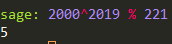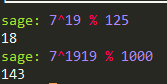(1) 手动计算 $2000^{2019} (mod\ 221)$

221 = 17*13

$2000^{2019} mod\ 221 \cong ([11^{2019} mod\ 17],[11^{2019}mod\ 13])$

$11^{\phi (17)} = 11^{16} ≡ 1\ mod\ 17$

$∴ 11^{2019} = 11^{16*126+3} ≡ 11^{3} ≡ 5\ (mod\ 17)$

$11^{\phi (13)} = 11^{12}≡ 1\ mod\ 13$

$∴ 11^{2019} = 11^{12*168+3} ≡ 11^{3} ≡ 5\ (mod\ 13)$

$x ≡ 5\ (mod\ 17)$

$x ≡ 5\ (mod\ 13)$

(由于式子很特殊这一步多此一举，但是为了鲁棒性我还是写了)

$x ≡ 5\ mod\ 17*13$

$\therefore 2000^{2019} (mod\ 221) = 5$(2) 7^{1919}的最后三位是多少?

1000 = 125*8

$7^{1919} mod\ 1000 \cong ([7^{1919} mod\ 125],[7^{1919} mod\ 8])$

$7^{\phi(125)} = 7^{5^3 - 5^2} = 7^{100} ≡ 1\ mod\ 125$

$∴ 7^{1919} mod\ 125 = 7^{19} mod\ 125$

$∴7^{19} = 7*(343^{6}) ≡ 7*((-32)^6) ≡ 7*(1024^3) ≡ 7*24^3 ≡ 18\ (mod\ 125)$

$7^{1919} ≡ (-1)^{1919} ≡ -1\ (mod\ 8)$

$x ≡ -1\ mod\ 8$

$x ≡ 18\ mod\ 125$

$x ≡ 143\ (mod\ 125*8)$

$∴7^{1919} mod\ 1000 = 143$